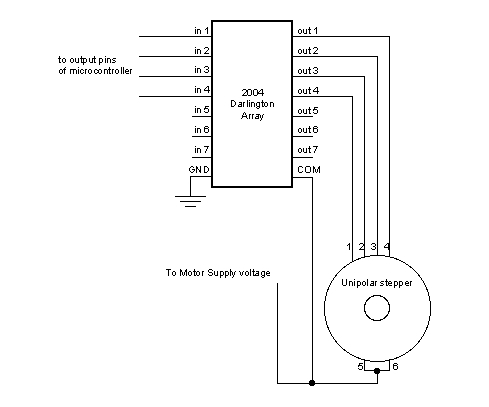# Reverse turning of Stepper motor

I have a stepper motor connected to my Arduino like this using the ULN2003A Darlington Array:(Ignore the potentiometer)

And I have programmed it with the following code:

``````#include <Stepper.h>

int in1Pin = 22;
int in2Pin = 23;
int in3Pin = 24;
int in4Pin = 25;

Stepper motor(512, in1Pin, in2Pin, in3Pin, in4Pin);

void setup()
{
pinMode(in1Pin, OUTPUT);
pinMode(in2Pin, OUTPUT);
pinMode(in3Pin, OUTPUT);
pinMode(in4Pin, OUTPUT);

motor.setSpeed(25);
}

void loop()
{
int steps = 360;

motor.step(steps);
delay(500);
}
``````

At the moment the motor rotates clockwise, how could I have it so it rotates in the opposite direction?

My code was copied and edited from here.

The problem was that I had the two middle wires the wrong way round as said here: http://forum.arduino.cc/index.php?PHPSESSID=kvi8dt2b5en5hhk02dlmjrotl5&topic=143276.msg‌​

The answer is simple. Just pass a negative number of steps as an argument to `motor.step();`.

Another note: You forgot a semicolon on your second to last line. IIRC this doesn't matter in C, but it's just bad practice to do this. If you add a line of code below that, then it won't work.

Example code:

``````#include <Stepper.h>

int in1Pin = 22;
int in2Pin = 23;
int in3Pin = 24;
int in4Pin = 25;

Stepper motor(512, in1Pin, in2Pin, in3Pin, in4Pin);

void setup()
{
pinMode(in1Pin, OUTPUT);
pinMode(in2Pin, OUTPUT);
pinMode(in3Pin, OUTPUT);
pinMode(in4Pin, OUTPUT);

motor.setSpeed(25);
}

void loop()
{
int steps = 360;
motor.step(steps);
delay(100);

steps = -360;
motor.step(steps);